Deflection of Beams

# Deflection of Beams Notes | Study Mechanical Engineering SSC JE (Technical) - Mechanical Engineering

## Document Description: Deflection of Beams for Mechanical Engineering 2022 is part of Strength of Materials (SOM) for Mechanical Engineering SSC JE (Technical) preparation. The notes and questions for Deflection of Beams have been prepared according to the Mechanical Engineering exam syllabus. Information about Deflection of Beams covers topics like and Deflection of Beams Example, for Mechanical Engineering 2022 Exam. Find important definitions, questions, notes, meanings, examples, exercises and tests below for Deflection of Beams.

Introduction of Deflection of Beams in English is available as part of our Mechanical Engineering SSC JE (Technical) for Mechanical Engineering & Deflection of Beams in Hindi for Mechanical Engineering SSC JE (Technical) course. Download more important topics related with Strength of Materials (SOM), notes, lectures and mock test series for Mechanical Engineering Exam by signing up for free. Mechanical Engineering: Deflection of Beams Notes | Study Mechanical Engineering SSC JE (Technical) - Mechanical Engineering
 1 Crore+ students have signed up on EduRev. Have you?

DEFLECTION OF BEAMS

The amount by which a beam deflects depends upon its cross-section and the bending
moment. For modern design there are two governing criteria viz. strength and stiffness.
• As per the strength criterion, the beam should be strong enough to resist bending
moment and shear force or in other words beam should be strong enough to resist
the bending stresses and shear stresses. And as per the stiffness criterion of the
beam design, which is equally important, it should be stiff enough to resist the deflection
of the beam or in other words the beam should be stiff enough not to deflect more
than the permissible limit. It means serviceability condition must be satisfied.
METHODS FOR SLOPE AND DEFLECTION AT A SECTION
1. Double Integration method

• This method is suitable for simple loading in simply supported beams and
• The double integration method is a powerful tool in solving deflection and slope of a beam at any point because we will be able to get the equation of the elastic curve.
In calculus, the radius of curvature of a curve y = f(x) is given by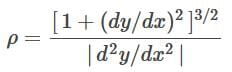In the derivation of flexure formula, the radius of curvature of a beam is given as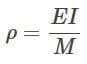Deflection of beams is so small, such that the slope of the elastic curve dy/dx is very small, and squaring this expression the value becomes practically negligible, hence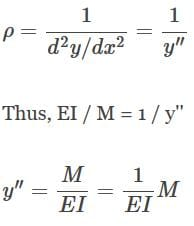If EI is constant, the equation may be written as: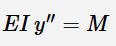where x and y are the coordinates shown in the figure of the elastic curve of the beam under load, y is the deflection of the beam at any distance x. E is the modulus of elasticity of the beam, I represent the moment of inertia about the neutral axis, and M represents the bending moment at a distance x from the end of the beam. The product EI is called the flexural rigidity of the beam.
2. Macaulay’s method
• This is a convenient method for beams subjected to point loading or in general
discontinuous loads or beams subjected to couples (concentrated moments).
• This method is similar to the double integration method but specialty of this
method lies in the manner in which the bending moment at any section is expressed
and in the manner in which integration is carried out.
3. Moment Area Method
• This method is suitable for cantilevers and simply supported beams carrying
symmetrical loadings and beams fixed at both ends i.e., those beams for which
the area and C.G. of area of B.M.D. can be found easily. This means this method
for non prismatic bars.
• This method is suitable for
(i) Cantilevers (because slope at the fixed end is zero).
(ii) Simply supported beams carrying symmetrical loading (slope at mid span is
zero).
(iii) Beams fixed at both ends (slope at each end is zero).
Note:
In moment area method continuity of slope is assumed so it is not applied when
internal hinges are present.

PROPERTIES OF PLANE AREAS
Notation : A = area
x, y = distances of centroid C
Ix, Iy = moments of inertia with respect to the x and y axes respectively.
Ixy = moments of inertia with respect to the x and y axes
Ip = Ix + Iy = polar moment of inertia
IBB = moment of inertia with respect to axis B-B.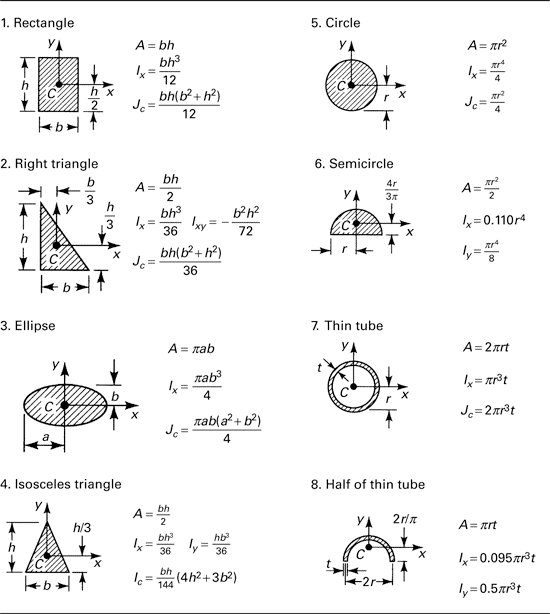4. Conjugate Beam Method
• This method is convenient if flexural rigidity of the beam is not uniform throughout
the length of the beam. This method is suitable for beams carrying internal hinge.
An imaginary beam for which the load diagram is EI,M diagram, of the givenbeam is called the conjugate beam.
(i) The slope at any section of the given beam is equal to the S.F. at the
corresponding section of the conjugate beam i.e. SFD of conjugate beam is
slope curve of real beam.
(ii) The deflection at any section for the given beam is equal to the bending moment
at the corresponding section of the conjugate beam. i.e. BMD of conjugate
beam is deflection curve of real beam.
Important points :
(i) A stable and statically determinate real beam will have a conjugate beam which
is also stable and statically determinate.
(ii) A unstable real beam will have statically indeterminate conjugate beam, hence if
a conjugate beam is found to be statically indeterminate, it is concluded that the
real beam is unstable and further analysis is not appropriate.
(iii) Statically indeterminate real beam will have unstable conjugate beam hence its
conjugate load must be such that it maintains equilibrium.
5. Method of Superposition
It is suitable for cantilevers containing concentrated loads & concentrated moments.
This method can also be used for non prismatic bars i.e. varying EI(Flexural rigidity).
6. Strain Energy method (Castigliano’s theorem)
It is suitable for cantilevers and beams having varying EI or varying depth of beam.
This methods is very useful in case of determinate frames and arches. This can also
be used when internal hinge is provided.

The unit-load method is a technique that will help us to quantify displacements and rotations of the equilibrium configuration, that is, the shape of the structure after it has managed to equilibrate the applied loads.
Method used to find deflection of truss joints (perfect frames) : STUDENT CORNER
(i) Unit load method (Maxwell’s method)
(ii) Castigliano’s theorem (Strain energy method)
(iii) Graphical method (Williot Mohr diagram)
(This method is used for trusses only & cannot be used for beams)
STANDARD CASES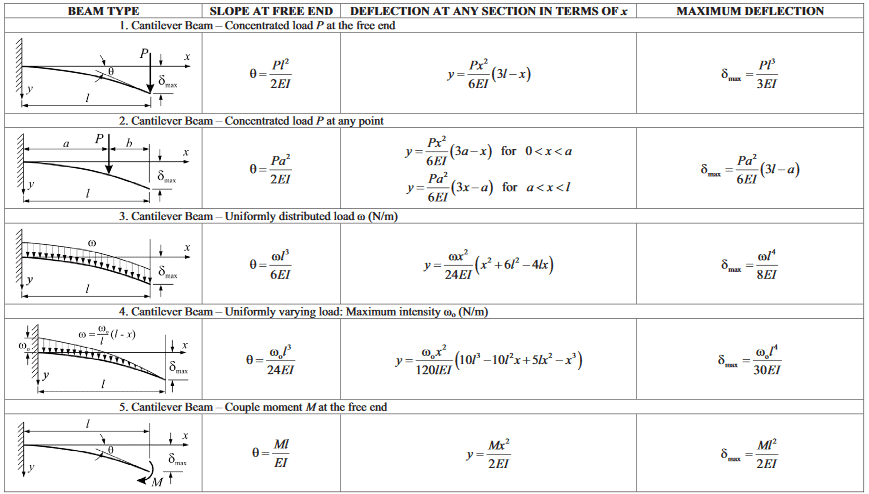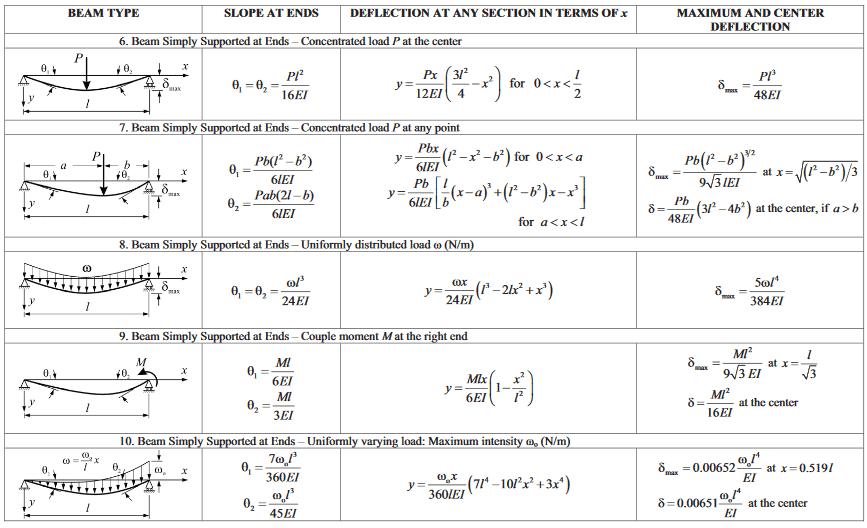The document Deflection of Beams Notes | Study Mechanical Engineering SSC JE (Technical) - Mechanical Engineering is a part of the Mechanical Engineering Course Mechanical Engineering SSC JE (Technical).
All you need of Mechanical Engineering at this link: Mechanical Engineering

## Mechanical Engineering SSC JE (Technical)

6 videos|89 docs|55 tests
 Use Code STAYHOME200 and get INR 200 additional OFF

## Mechanical Engineering SSC JE (Technical)

6 videos|89 docs|55 tests

Track your progress, build streaks, highlight & save important lessons and more!

,

,

,

,

,

,

,

,

,

,

,

,

,

,

,

,

,

,

,

,

,

;